Excel Tips & Tutorials:
Multiple Regression
 Suggest a Link Alphabetize Page Printer-Friendly List
Watch and Learn
Watch and Learn
Watch and Learn
Watch and Learn
 Regression with Multiple Dummy Variables and Interaction Terms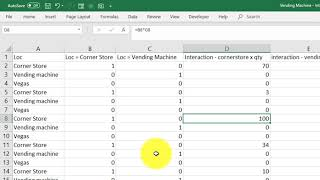Alym Amlani demonstrates with simple business example (8:11)Multiple Regression Analysis with Data Analysis ToolPak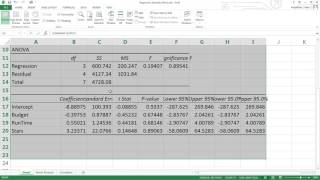Tobin Porterfield provides example with movie data (4:56)Graphing Two Data Series on the Same Scatterplot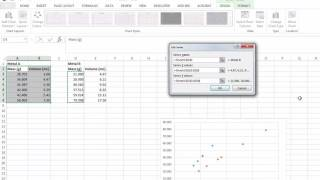Plot two data sets on the same scatterplot for visual comparison (6:07).Conducting a Multiple Regression using Data Analysis Tools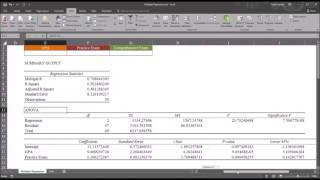From Todd Grande (10:14).Regression with Multiple Dummy Variables and Interaction TermsAlym Amlani demonstrates with simple business example (8:11)Multiple Regression Analysis with Data Analysis ToolPakTobin Porterfield provides example with movie data (4:56)Graphing Two Data Series on the Same ScatterplotPlot two data sets on the same scatterplot for visual comparison (6:07).Regression with Multiple Dummy Variables and Interaction TermsAlym Amlani demonstrates with simple business example (8:11)Multiple Regression Analysis with Data Analysis ToolPakTobin Porterfield provides example with movie data (4:56)Regression with Multiple Dummy Variables and Interaction TermsAlym Amlani demonstrates with simple business example (8:11)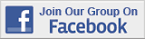Over 18,000 Members!